# Birds

On the farm they have a total of 110 birds. Geese and turkeys together is 47. Hens is three times more than the turkey. How much is poultry by species?

h =  26
k =  21
s =  63

### Step-by-step explanation:

h+k+s = 110
h+k = 47
s = 3 k

h+k+s = 110
h+k = 47
s = 3•k

h+k+s = 110
h+k = 47
3k-s = 0

h = 26
k = 21
s = 63

Our linear equations calculator calculates it.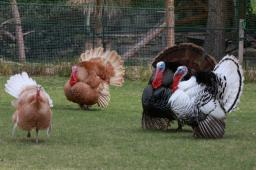Did you find an error or inaccuracy? Feel free to write us. Thank you!Tips to related online calculators
Do you have a linear equation or system of equations and looking for its solution? Or do you have a quadratic equation?

## Related math problems and questions:

• Farm2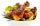The farm bred turkeys, geese, hens, and chickens. Everything is 400. Turkeys and geese a hen are 150. No hen has more than one chicken, but some hens have no chick. Half of them are also a quarter of the chickens. When the turkey was subtracted by 15, we
• The same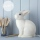The same number of rabbits and hens are running on the farm. 468 feet run around the yard. How many rabbits and hens do they have on the farm?
• Dividing moneyVilem, Cenek, and Edita divided the money they earned by spreading the leaflet. Vilem got 240 CZK more than Cenek and twice more than Edita. Edita got 400kc less than Vilem.
• Buns and toastsTwo buns weigh 10 grams more than two toasts. One Bun and two Toast weigh a total of 110 grams. How many grams weigh three toasts? How many grams does a Bun weigh?
• Honza + Alice + Tonda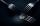Honza + Alice + Tonda have a total of 111 USD. The ratio between Honza and Alena is 5:6 and the ratio between Alice and Tonda is 4:5 How many money have each of them?
• GardensThe garden has the square shape with circumference 124 m. Divide it into two rectangular gardens, one should circumference 10 meters more than the second. What size will have a gardens?
• Cents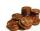Julka has 3 cents more than Hugo. Together they have 27 cents. How many cents has Julka and how many Hugo?
• The store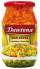The store received the same number of cans of peas and corn. The first day sold 10 cans of peas and 166 cans of corn to leave 5 times more peas than corn cans. How many cans of each kind were in the store?
• Chocolate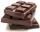How many times do we have to break a chocolate bar composed of 10 × 12 pieces to get the 120 parts?
• Students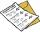The class has 22 students. 2 of which have assessments of 2. The count of assessment of 1 is three times more than the count of students with an assessment of 3. How many students have an assessment of 1, and how many students have an assessment of 1.
• Rabbit family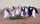Rabbit family ate 32 pieces of carrots, small one ate 6 pieces, dad 5 more than the mother. How much ate mom?
• TrioTrio of workers earn 750 euros. Money divided by the work that each of them made​​. First received twice as the second, the second received three times more than the third. How many got everyone from workers?
• Rabbits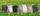In the hutch are 48 mottled rabbits. Brown are 23 less than mottled and white are 8-times less than mottled. How many rabbits are in the hutch?
• WineA bottle of wine costs 21 euros, and wine is 20 times more expensive than a bottle. How much does a bottle cost?
• Six hensSix hens weigh 3 kg more than three geese and 7 kg less than five geese. How much does a hen weigh, and how many geese?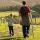Daddy is a 46-year-old, and his son is at the age of 16. When (which year) was/will daddy five times more years than his son?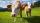On the farm were housed 70 heads of cattle with food supply for 180 days. After 60 days, 10 head of cattle sold, and for the next 30 days they sold 10 more. How many days is sufficient supply of feed for the remaining cattle?